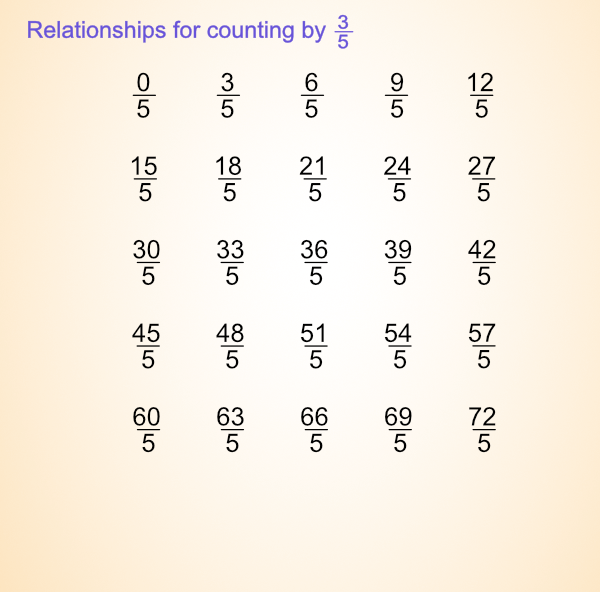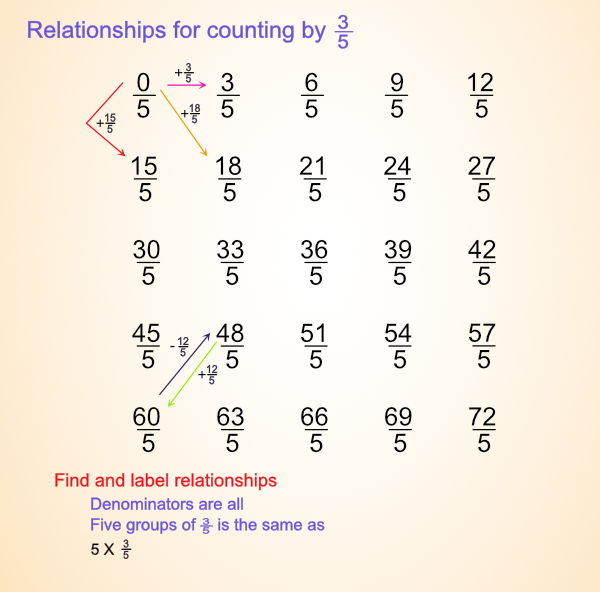# Fractions: Counting by three-fifths

We don’t need no more rappers don’t need no more basketball players, no more football players. We need more thinkers. We need more scientists. We need more managers. We need more mathematicians. We need more teachers.
WE NEED MORE PEOPLE WHO CARE.
Tupac Shakur

Challenge:

Count by 3/5's and explore patterns in the sequence of groups of three-fifths.Hint:

1. Count by 3/5s.
2. Make a list of groups of three-fifths.
3. What do you notice about the numbers?
4. Organize them into rows with each row starting with a whole number equivalent.
5. What patterns do you notice?

Discussion:

Count orally: three-fifths, six-fifths, nine-fifths, twelve-fifths, fifteen-fifths, eighteen-fifths, ...

Record the group and number of fifths in each group ...

 Number of groups Total 0 1 2 3 4 5 6 7 8 9 10 11 12

 Number of groups Total 13 14 15 16 17 18 19 20 21 22 23 24 25

Patterns for the denominator ...

Patterns for the numerator ...

Group ...

What patterns can you find when you arrange them in a grid starting a new row for each whole number equivalent. Like below.## Sample with some notesSome patterns:

• Denominators are all fifths.
• Numerators ar 0, 3, 6, 9, 12, ...
• Numerators add three each tme.
• Numerators are multiple of three.
• Numerators are odd, even, odd, even, ...
• You add 15 going down each column.
• Five groups of three (15/5) are the same as three.
• Ten groups of 3/5 are the same as six.
• Number in ones place in each column alternate. (0,5; 3,8; 6,1; 9,4; 2,7).

What will a graph look like for the relationship for groups and number of fifths?

What will be the difference if the number of groups are graphed as x-values and totals as y-values vs. the number of groups as y-values and totals as x-values.

## GraphTop